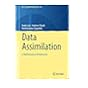Normal view MARC view ISBD view

# Methods of mathematical finance

Material type:BookPublisher: Berlin Springer-Verlag 1998Description: 407 p.ISBN: 0387948392.DDC classification: 511.8
Tags from this library: No tags from this library for this title.511.8 G3N2 The nature of mathematical modeling 511.8 H2F4 Finite Markov chains and algorithmic applications 511.8 I5M2 Mathematical modelling: a case studies approach 511.8 K2M3 Methods of mathematical finance 511.8 L2D2 Data assimilation: a mathematical introduction 511.8 M2K6 Knapsack problems: algorithms computer implementations 511.8 M3C6 Concepts of mathematical modeling